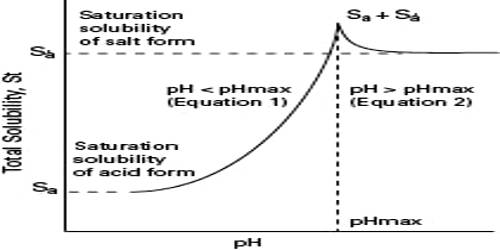Chemistry

# Effect of pH on SolubilityEffect of pH on Solubility

When one or more of the ions in a solubility equilibrium is derived from a weak acid or a weak base, the solubility of the compound is profoundly affected by pH. For example, let us consider the case of Mg(OH)2. The solubility equilibrium for this hydroxide is

Mg(OH)2 + aq ↔ Mg2+ (aq) + 2OH (aq)

The value of Ksp for Mg(OH)2 is 1.8 x 10-11. Suppose that solid Mg(OH)2 is placed in a buffer solution of pH = 9. The solution has a pOH of 5. i.e., in the solution [OH] = 1 x 10-5. Inserting this value of [OH] in the expression of the solubility product-

Ksp = [Mg2+] [OH]2 = 1.8 x 10-11

= [Mg2+] [1.8 x 10-11] = 1.8 x 10-11

= [Mg2+] = 1.8 x 10-1 = 0.18 mol L-1.

Thus Mg(OH)2 is quite soluble in slightly alkaline solution. If the solution was made more acidic the [OH] will decrease and the solubility will increase because the [Mg2+] has to increase in order to maintain equilibrium.

On the contrary, an addition of H3O+ to a saturated solution at a compound with an anion of the strong acid will have no effect on the equilibrium position. As an example, let us consider the following equilibrium:

AgCl(s) ↔ Ag+ (aq) + Cl (aq)

Here, Cl ion, a conjugate base of a strong acid, HCl, can co-exist in solution with high H3O+. The Cl ion does not leave the solution, so equilibrium position is not affected.